Introduction

Volatility is a key feature of financial markets. It is commonly used as a measure for risk and is a common an indicator of the investors’ fear and concern about the future. While higher volatility means bigger profits and losses in a directional strategy (one which bets on a future movement of the price in a certain direction), non-directional strategies make profits from the changes in volatility itself, either if the market is bullish or bearish. The most straightforward strategies involve derivatives on indexes which follows a certain asset’s volatility. A well-known example are the derivatives built on the VIX, an index released by the CBOE representing the “market expectations of near-term volatility conveyed by S&P 500 stock index option prices” (source: CBOE.com).

Formal derivation of the variance portfolio

Instead of using a derivative on a volatility index, an alternative strategy is trading a portfolio, the “variance portfolio”, whose value is equal to the expected future variance (the squared expected volatility) of a certain underlying (for example, an equity index) and whose change in value is equal to the change in expected variance. The formal derivation of this portfolio is based on the following assumptions:

– the market is frictionless;

– the underlying pays no dividends;

– the time evolution of the underlying price at time t St follows a geometric Brownian motion with drift (St,t) and volatility (St,t) both dependent on time and underlying price (even when the explicit dependence will be omitted) Eq.1.It must be noted that these assumptions are weaker that the ones of the Black-Scholes model. The average variance over the time interval from 0 to T in continuous time is given by the integral average in Eq.2To obtain the integrand in Eq.2, you use Ito’s lemma to derive the SDE in terms of the logarithm of St Eq.3 and subtract Eq.1 from it. The result can be rearranged into Eq.4 which does not depend explicitly on the drift  and the Wiener process dWt.The SDE is solved integrating over the time interval from 0 to T to obtain Eq.5The right hand term is the integrand we need to obtain the future variance, while the left hand term is the value of a portfolio consisting in:

– a continuously rebalanced long position in the underlying so that, at every time t, the portfolio is long 1/St shares (it is the same as keeping 1€ invested in the underlying);

-a short position in an exotic derivative on St, called “log-contract”, which at T pays the logarithm of the underlying, divided by the price at 0. The value of this portfolio will be equal to the realized variance over the considered time interval, as long as it is continuously rebalanced.

For the following steps, we will take expectations under the risk-neutral probability measure Q (the probability measure implied by the market so that the price of a financial instrument is the present value of the expected future cash flows). If the risk free interest rate r with maturity T is constant, under Q the drift in Eq.1 is equal to r and Eq.1 leads to Eq.6.Less trivial is the derivation of the log-contract. First of all, as S0 is a constant, the expression can be rewritten as Eq.7, where S* is a freely chosen constant (which will be useful later).The payoff of the first term in the right hand term in Eq.7 is shown in Fig.1. This payoff can be split into a short position in a forward contract (blue line) and a “curved straddle” (red line).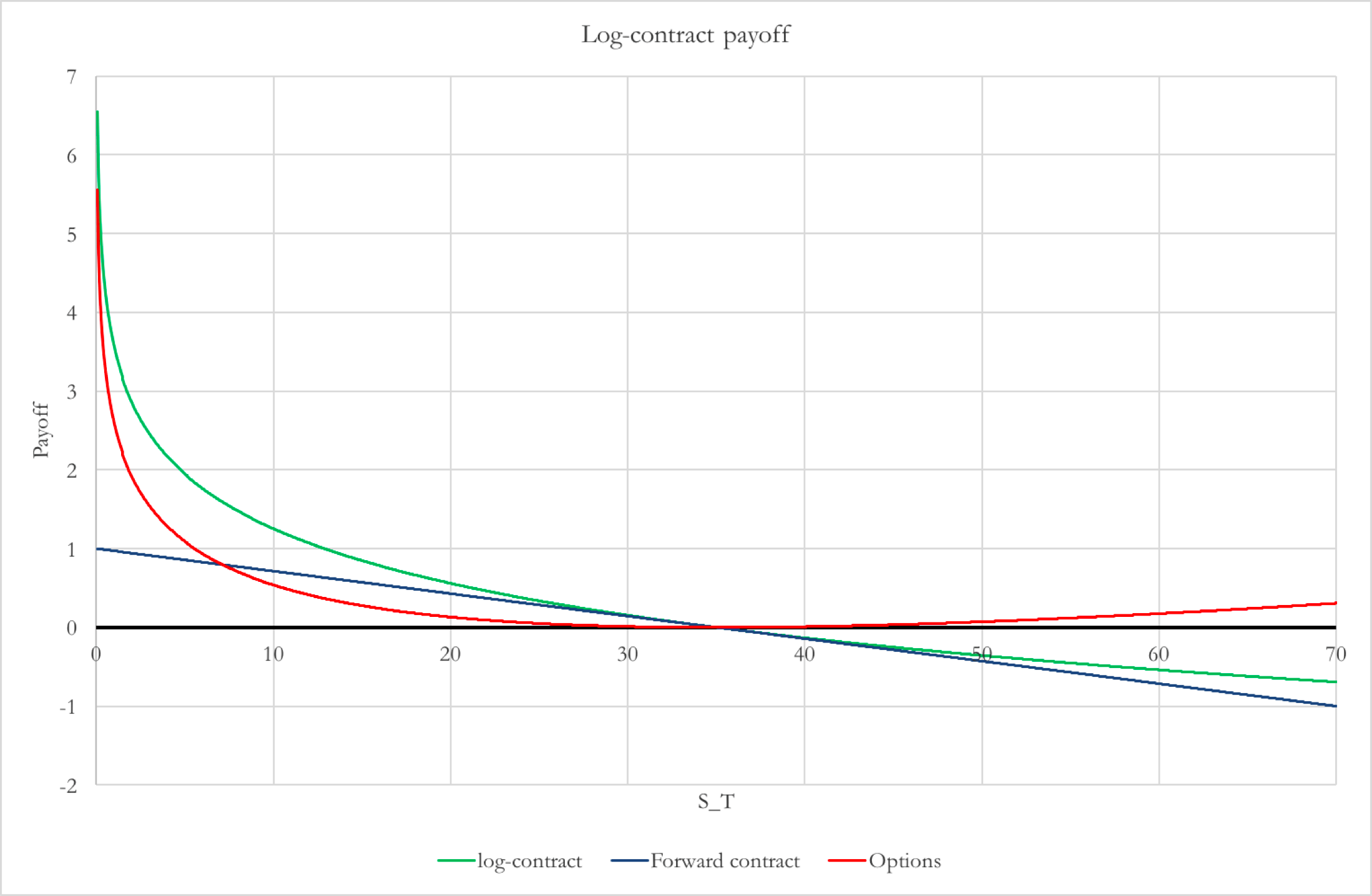Fig. 1 An example of log-contract payoff

Assuming that there are infinitely many options with strikes covering all possible values, there is a set of weights w(K), dependent on the strike price, such that a portfolio of OTM puts and calls with this set of weights has the same payoff as the “curved straddle”. It can be mathematically proven thatEq.7 can be rewritten as Eq. 9The practical meaning of Eq. 9 is that we can replicate the pay-off of an exotic derivative combining:

– a position (long or short depending on the relative position of S0 and S*) in a risk-free asset;

– a short position in 1/S* forward contracts;

– a long position in 1/K2 put plain vanilla options, for all strikes K from 0 to S* and expiring in T;

– a long position in 1/K2 call plain vanilla options, for all strikes K from S* to infinite and expiring in T.

Putting the results of Eq.9 into Eq.5, taking the expected value under Q on both sides, we get a formula for the expected variance E[V] Eq.10The financial meaning of Eq. 10 is clearer if we substitute the discounted value of the expected payoff of an instrument with its price in 0 and summarize some terms as in Eq. 11.Where Prf is the price in 0 of a risk-free asset which pays 1€ in T, F(S*) is the price of a forward contract with delivery price S*, C(K) and P(K) are respectively the price of a call and put options with strike price K. Eq. 10 becomesnrf, nF and nO(K) are respectively the number of risk-free assets, forward contracts and options which constitute the variance replicating portfolio.

Constant Vega: a Black-Scholes digression

The position in infinitely many options with weight inversely proportional to the strike price can be visualized with the help of the greeks. The “greeks” of an option are the ratio between the change of the option value and the infinitesimal change of a variable which determines the option value. A simple way to determine greeks is to derive the Black-Scholes formula for the option value with respect to one of the price determinants: such derivative is the corresponding greek. Here, we are interested in the change in value with respect to changes in variance, which is the “Variance Vega”. Eq.13 is the explicit Black-Scholes formula for the Variance Vegawhere  is the Gaussian probability density distribution.

Fig. 2 shows the value of VVar for different underlying prices S0. If the option is ATM, it is very sensible to changes in variance, but the more the underlying changes values, the less a change in variance will affect the priceFig. 2 Value of VVar for different S0

The portfolio we seek must have a uniform VVar, so that its behavior is not affected by the level of the underlying. Combining options with different strikes into a portfolio improves the VVar distribution, Fig.3.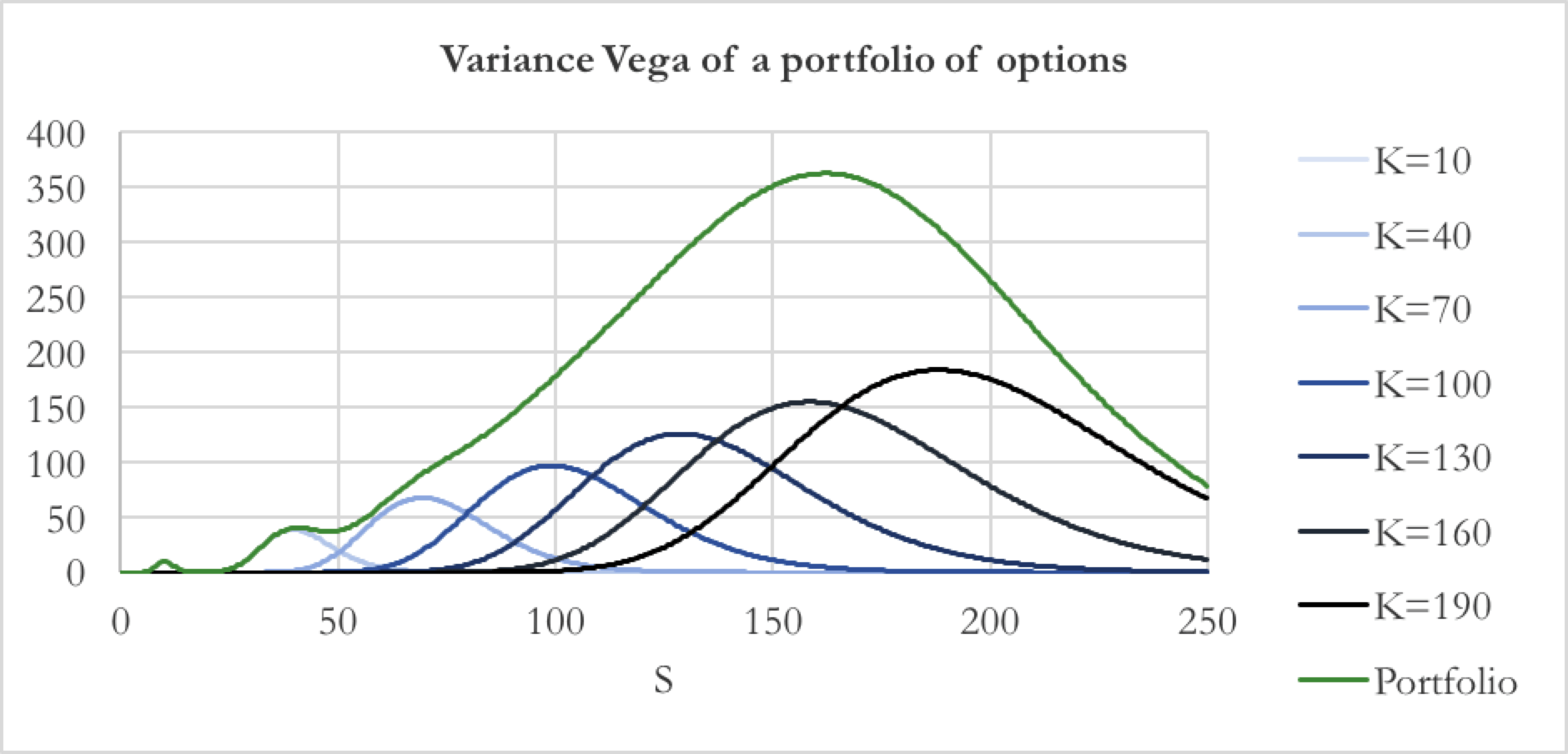Fig. 3 Value of VVar of different options and of the portfolio combining them

The distribution is flatter, but asymmetric because the maximum Variance Vega increases with strike price and has a wave pattern on the left side because of the distance between strikes. These defects are overcome using the weight nO(K) in Eq. 11.b in our portfolio and increasing the frequency of strikes (to the limit of infinity). The result is Fig.4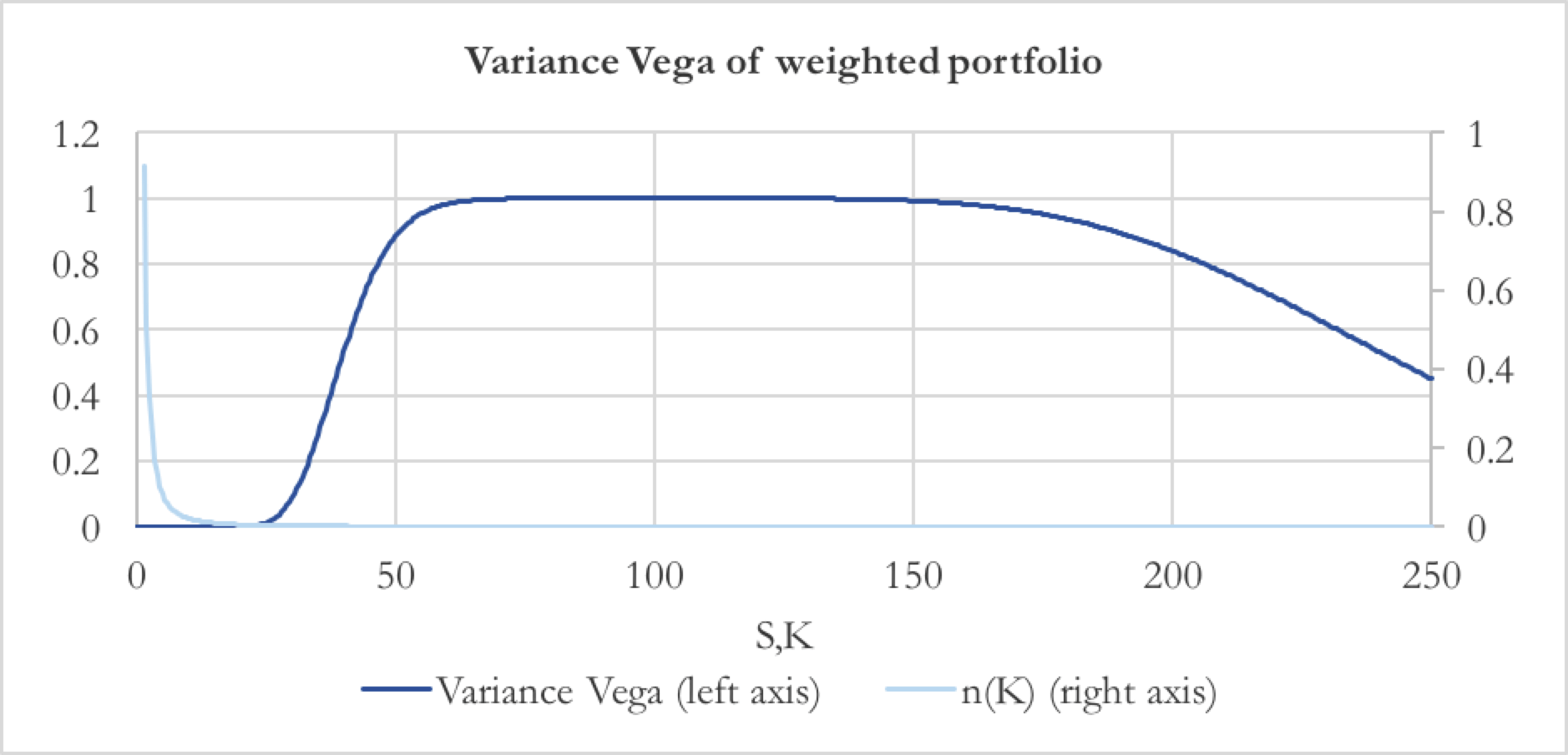Fig. 4 VVar distribution of the portfolio with weight nO

Here, we used options with strike from 40 to 245 with difference =0.5 between them. The central region of the distribution is almost flat, which means that whatever the value of the underlying in this region, the effect of a change in variance to the portfolio is same. In particular, the value of VVar in this region is 1, so for every change in variance, the value of the portfolio changes exactly by the same amount (we shall remember that the VVar of the other components of Eq.12, the risk-free asset and the forward, is null). This feature makes it an optimal strategy for an investor seeking exposure to the variance of an asset.

A practical example: building the variance portfolio on the S&P 500

Eq. 12 is at the base of the algorithm used by the CBOE to calculate the VIX index from the options on the S&P 500 equity index. So, here we build our portfolio on the S&P 500 in order to check if its value is coherent with the VIX quotation. We retrieve the current S&P 500 figure (S0 = 2368.64) and the option chain on the S&P 500 expiring in 28 days from the CBOE.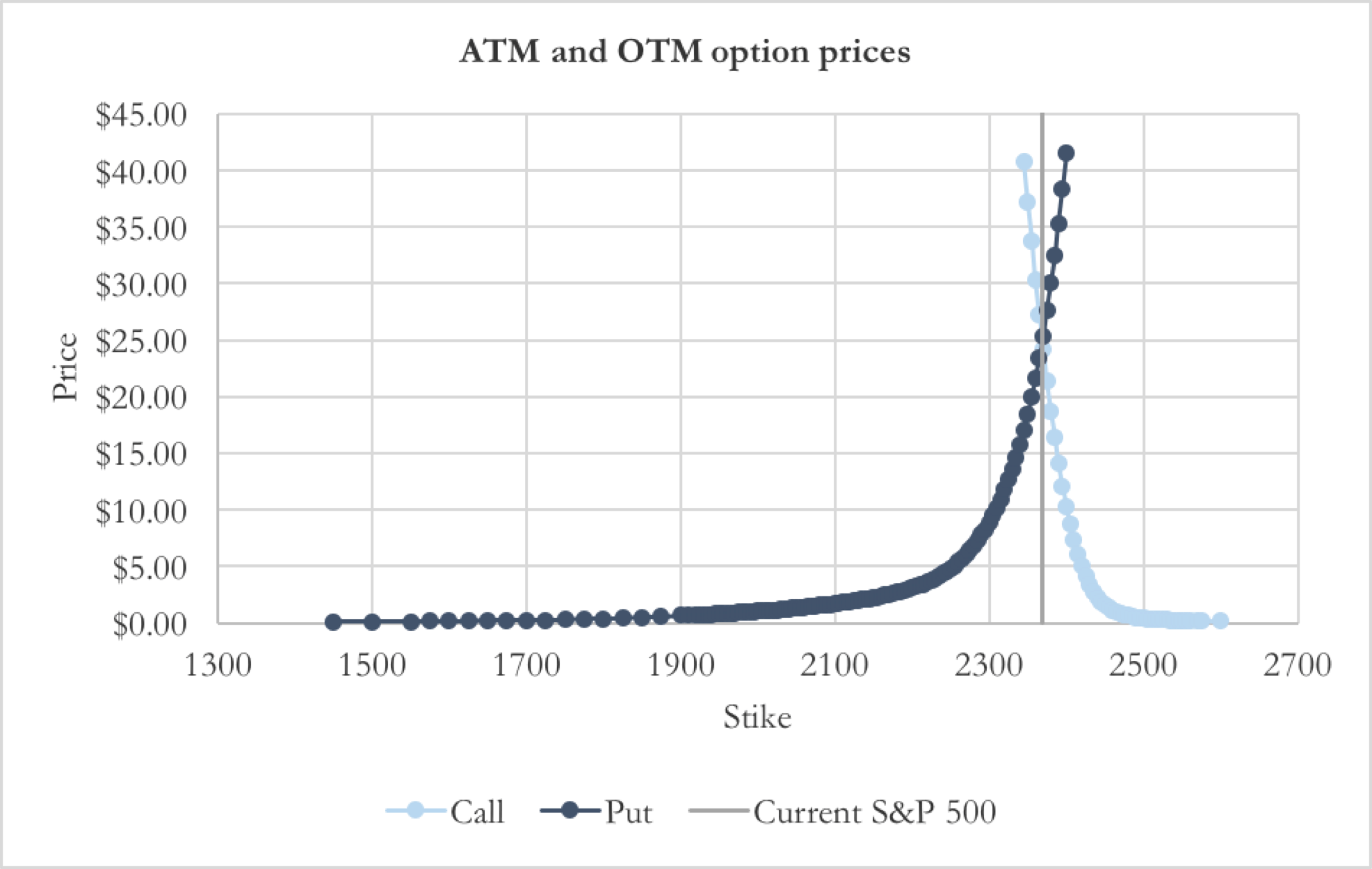Fig.5 Prices of ATM and OTM options on the S&P 500 (source: CBOE.com)

Only the most liquid options will be used for the calculation, so we drop the illiquid ones and choose S* as the closest strike to the future price of the underlying, in this case S*=2370, so that the portfolio will be formed by OTM options. Because there is a limited number of strikes, we have to approximate the integral in Eq. 14 with a sum over the strikes and an appropriate number of each option. In particular, we want to replicate the pay-off functionWith call option strikes KiC and put option strikes KjP ordered asOne way of approximating f(ST) is decomposing it into segments connecting f(K) valuated at the different available strikes, as in Fig. 6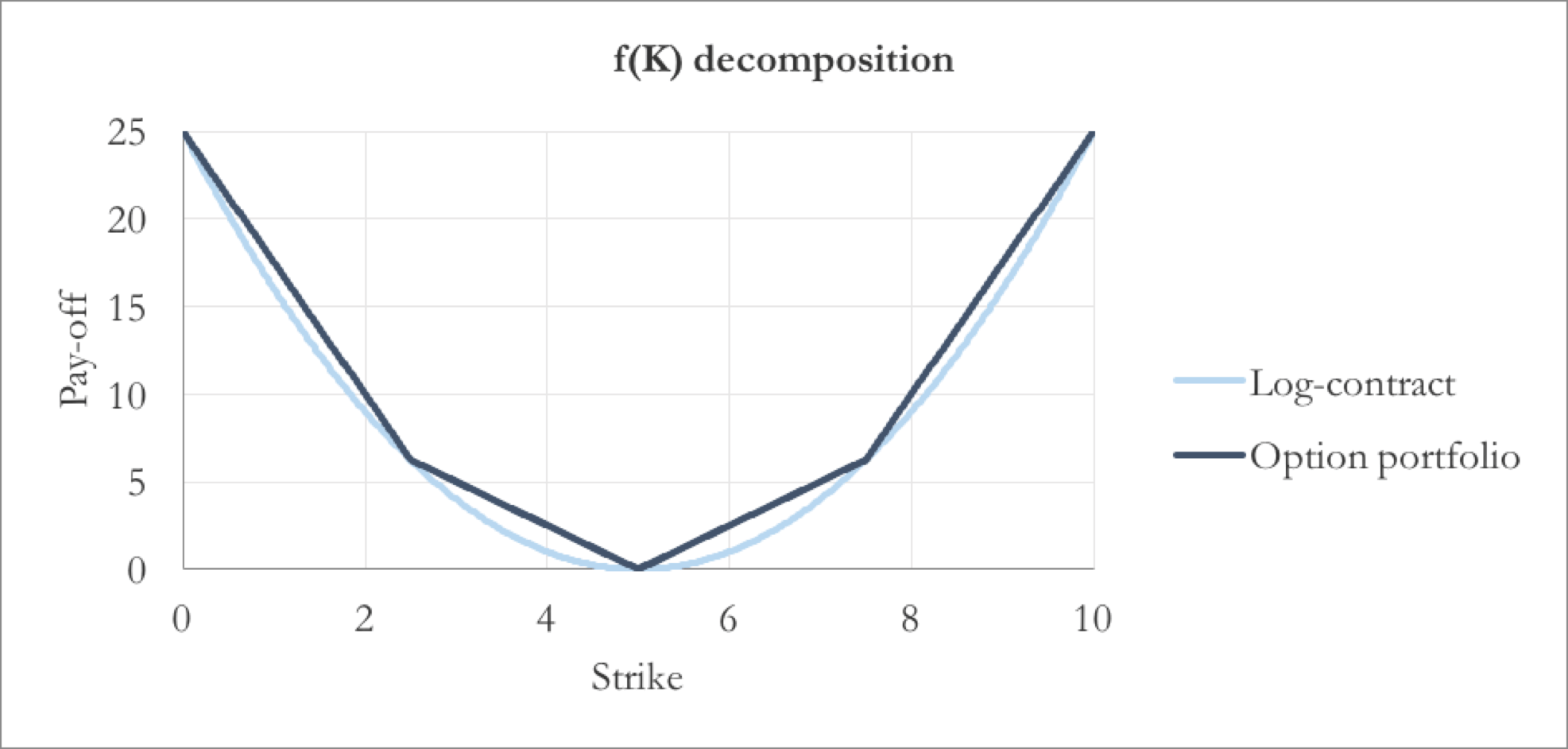Fig.6 A graphic example of the segment decomposition

f(ST) is convex, so our approximation always lies above it, thus the value of our portfolio will overestimate the theoretical expected variance. nO is different from put to call options and is calculated recursively starting from K=S*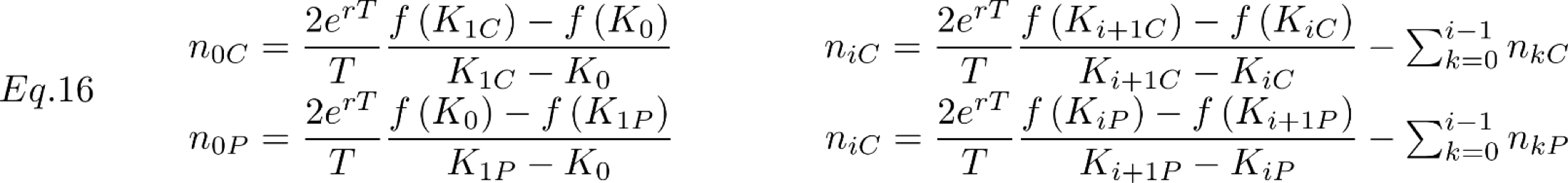The price of the risk-free asset and of the forward contract are calculated as their fair price under the risk-neutral probability measure.The values needed in Eq.12 are reported in Table 1. The expected variance represented by our portfolio value is 0.0157 and the expected volatility is 12.5%, which is consistent with the VIX closing value of 12.37. The difference can be explained by the fact that our portfolio is the 28-day variance, while the VIX is calculated averaging the expected volatilities of different time horizons to get the 30-day figure).

Table 1 Value of the components of the portfolio on S&P 500

 Risk-free asset price: \$ 0.99931 N risk-free: 0.00332 Forward price: \$ 0.27953 N forward: -0.01186 Value risk-free position: \$ 0.00331 Value forward position: \$ -0.00331 Value of option position: \$ 0.01633 Portfolio value: \$ 0.01633 Expected volatility: 12.78%

A bet on French elections: volatility of CAC 40

Trading the S&P 500 volatility is easy thanks to the widespread range of derivatives on the VIX and ETFs containing these derivatives, so the strategy presented above would hardly ever be implemented. However, it can be useful for other underlyings for whom there are few derivatives on volatility or there are none, as long as there are derivatives on the underlying itself.

Considering the uncertainties of French elections (which will take place from 23th April to 7th May) which are already the spread of French government bonds, we want to implement our strategy on the CAC 40 index, a capitalization-weighted benchmark representing the main stock exchanged in the Euronext Paris bourse. We choose the contracts expiring on the 19th May in order to be exposed to the volatility during and soon after the elections. The value of the index at t=0 S0 is 5083.07. In this case, we have an implied dividend yield q (continuously compounded) of 9.73%, so we have to modify eq 10 to take the dividend into account. It can be proven that the only change is the number of risk-free assets nrf and, of course, the formula to calculate the fair price of the forward contract, which become Eq. 18.Here the main issue is the small number of available strikes (9 strikes, raging from 4900 to 5300). We choose S*=5050, between the present value of CAC 40 and the expected value at T, so we will use 6 call options and 4 put options. Using Eq. 14 to express the pay-off we want to replicate and definingas the pay-off of the optimal portfolio replicating f(ST) (where wiC is the number of call option with strike KiC and wjP is the number of put option with strike KjP).

We want to find the wiC and wjP which minimize the error between f and frepl, which can be expressed asEq.20 is a continuous formulation of the least squares optimisation over the interval [Smin, Smax]. where Smin and Smax are chosen arbitrarily. Here we chose [Smin, Smax] so that, under the risk-neutral probability measure, the interval is centred at the expected value of CAC 40 in T and that the price in T will fall into this interval with 95% probability (in particular we used the implied volatility of the contract with 5050 strike price as a proxy for volatility). The interval is [4347, 5756]. After optimizing Eq. 20, f and frepl areFig. 7 The payoff replication using traded options (Source: Bloomberg)

we find the optimal number of option niC and njP asThe figures so obtained are shown in Table 2. Table 3 reports the value of the different constituents of the portfolio.

Table 2 Number of options per strike

 Strike N Options Put 4900: 1.259E-04 Put 4950: 8.195E-05 Put 5000: 1.388E-05 Put 5050: 4.633E-06 Call 5050: 2.741E-06 Call 5100: 0 Call 5150: 4.095E-05 Call 5200: 7.347E-05 Call 5250: 9.757E-05 Call 5300: 3.326E-06

Table 3 Value of the portfolio

 Risk-less asset price: € 1.0005 N risk-less asset: -0.1043 Forward price: € -35.2773 N forward: -0.0029 Value risk-free position: € -0.1044 Value forward position: € 0.1040 Value option position: € 0.0377 Portfolio value: € 0.0374 Expected volatility: 19.33%

With this information, we can calculate the number of riskless assets and of forward contracts forming the portfolio and calculate the total value of the portfolio (using the midpoint between the bid and ask for the option prices). The results are the following. It is interesting to compare the square root of the portfolio value 19.33%, which is the expected standard deviation of returns, with the implied volatility of ATM options.

Table 4 Implied volatilities on CAC 40

 Strike Implied volatility 5000 20.13% 5050 19.55% 5100 19.00%

We find that our variance-replicating portfolio is consistent with the implied volatilities. Eventually, we calculate the percentage of capital to be invested in each asset.

Table 4 Asset weight in the portfolio

 Asset Weight Risk-less asset: -279.43% Forward: 278.45% Call 5050: 0.94% Call 5100: 0.00% Call 5150: 8.78% Call 5200: 11.98% Call 5250: 11.69% Call 5300: 0.28% Put 5050: 1.99% Put 5000: 5.14% Put 4950: 26.04% Put 4900: 34.15%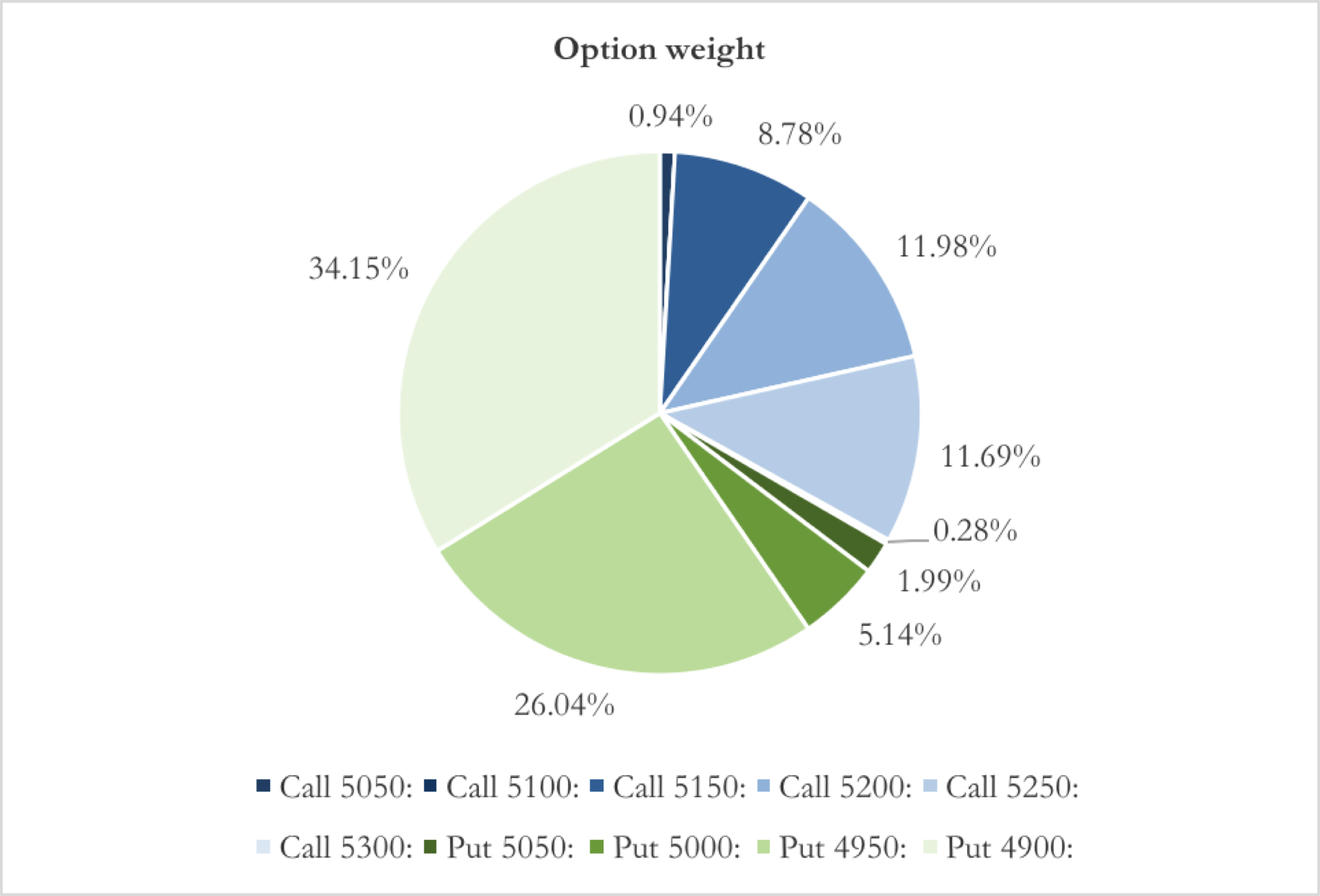Fig.8 Weight of each strike in the portfolio. Calls in blue, puts in green

The negative weight for the riskless asset means that we need to borrow near three times our capital at the risk free rate to implement our strategy.